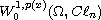Electron. J. Diff. Equ., Vol. 2012 (2012), No. 227, pp. 1-10.

### Weak solutions for A-Dirac equations with variable growth in Clifford analysis Binlin Zhang, Yongqiang Fu

Abstract:
In this article we show the existence of weak solutions for obstacle problems for A-Dirac equations with variable growth in the setting of variable exponent spaces of Clifford-valued functions. We also obtain the existence of weak solutions to the scalar part of A-Dirac equations in space.

Submitted November 18, 2012. Published December 17, 2012.
Math Subject Classifications: 30G35, 35J60, 35D30, 46E35.
Key Words: Clifford analysis; variable exponent; A-Dirac equation; obstacle problem.

Show me the PDF file (241 KB), TEX file, and other files for this article.Binlin Zhang Department of Mathematics Harbin Institute of Technology Harbin 150001, China email: zhangbinlin2012@yahoo.cn Yongqiang Fu Department of Mathematics Harbin Institute of Technology Harbin 150001, China email: fuyqhagd@yahoo.cn